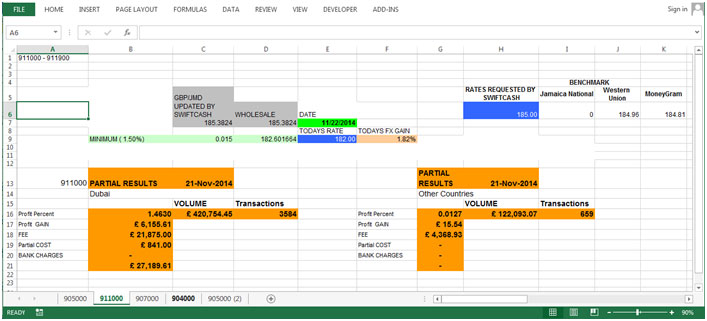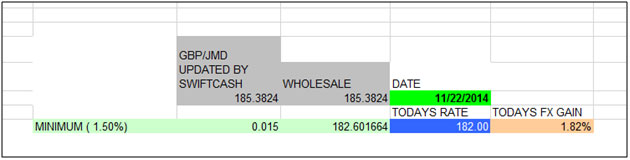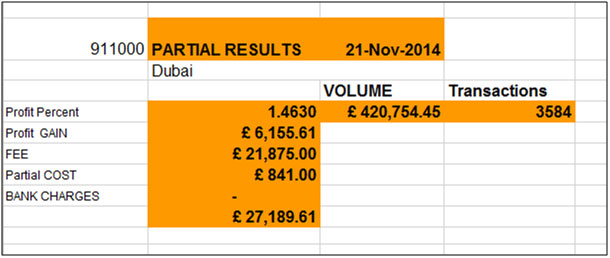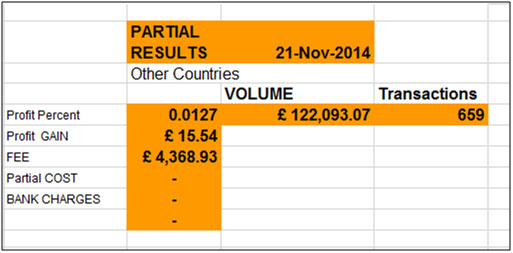# Customers’ Orders Book by gillani77

Created by: gillani77

In this article we will learn about how to prepare customer order book in Microsoft Excel 2013.

Customer’s order book used to calculate profit gain, profit percentage etc. information about the customers.

Let’s take an example to understand how we can prepare the Customers order book.In this table we can see the whole sale price and today’s price, according to these amount we can return the todays fix gain. In this report today’s fix gain amount will be equals to =1-(todays rate/whole sale rate).

Partial Result for DubaiIn this table we can return the customer’s amount status such as profit percent, profit gain, fee, partial cost, bank charges, volume and transactions.

Profit percentage will be = (Volume/Profit Gain)*100

Partial cost will be = ((Fee+Volume)/100)*0.19

Bank Charges will be = (Profit Gain + Fee)-Partial Cost

Partial Result for DubaiIn this table we can return the customer’s amount status such as profit percent, profit gain, fee, partial cost, bank charges, volume and transactions.

Profit percentage will be = (Volume/Profit Gain)*100

1.